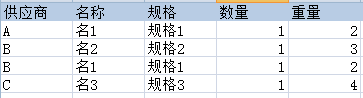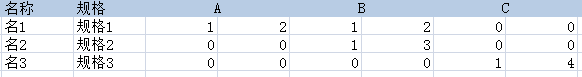###### cpfirst

2015-04-19 05:39 阅读 1.7k

# 求助：sql 列变行，列对多列的问题

5declare @sql varchar(8000)
set @sql = 'select 名称 ,规格'
select @sql = @sql + ', max(case 供应商 when ''' + 供应商 + ''' then 重量 else 0 end) [' + 供应商 + ']'
from (select distinct 供应商 from #tb) as a
set @sql = @sql + ' from #tb group by 名称 ,规格'
exec(@sql)

• 点赞
• 写回答
• 关注问题
• 收藏
• 复制链接分享

#### 4条回答默认 最新

• 已采纳
`````` create table 表(
供应商 varchar(20),
名称 varchar(20),
规格 varchar(20),
数量 int,
重量 int
);
insert into 表(供应商,名称,规格,数量,重量) values('A','名1','规格1',1,2);
insert into 表(供应商,名称,规格,数量,重量) values('B','名2','规格2',1,3);
insert into 表(供应商,名称,规格,数量,重量) values('B','名1','规格1',1,2);
insert into 表(供应商,名称,规格,数量,重量) values('C','名3','规格3',1,4);

SELECT 名称,规格
,SUM(CASE 供应商 WHEN 'A' then 数量 else 0 end) A数量
,SUM(CASE 供应商 WHEN 'A' then 重量 else 0  end) A重量
,SUM(CASE 供应商 WHEN 'B' then 数量 else 0  end) A数量
,SUM(CASE 供应商 WHEN 'B' then 重量 else 0  end) A重量
,SUM(CASE 供应商 WHEN 'C' then 数量 else 0  end) A数量
,SUM(CASE 供应商 WHEN 'C' then 重量 else 0  end) A重量
FROM 表 group by 名称,规格
``````
点赞 评论 复制链接分享
• `````` SELECT 名称,规格
,SUM(CASE 供应商 WHEN 'A' then 数量 else 0 end) A数量
,SUM(CASE 供应商 WHEN 'A' then 重量 else 0  end) A重量
,SUM(CASE 供应商 WHEN 'B' then 数量 else 0  end) B数量
,SUM(CASE 供应商 WHEN 'B' then 重量 else 0  end) B重量
,SUM(CASE 供应商 WHEN 'C' then 数量 else 0  end) C数量
,SUM(CASE 供应商 WHEN 'C' then 重量 else 0  end) C重量
FROM 表 group by 名称,规格
``````
点赞 评论 复制链接分享
• 谢谢，大家，供应商是无限制的，要动态的

点赞 评论 复制链接分享
• declare @sql varchar(8000)
set @sql = 'select 名称 ,规格'
select @sql = @sql + ', max(case 供应商 when ''' + 供应商 + ''' then 重量 else 0 end) as [' + 供应商 + ']'
+ ', max(case 供应商 when ''' + 供应商 + ''' then 数量 else 0 end) as [' + 供应商 + ']'
from (select distinct 供应商 from #tb) as a
set @sql = @sql + ' from #tb group by 名称 ,规格'
exec(@sql)

点赞 评论 复制链接分享